Next: Dynamic Stability Up: Associative Decorrelation Dynamics Previous: Associative Decorrelation Dynamics

## Dynamic Equations

The set of equations describing the change of the state of activity of the neurons is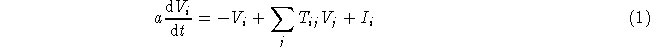in which a is a time constant,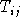is the strength of the synaptic connection from neuron j to neuron i, and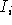is the additional feedforward input to the neuron besides those described by the feedback connection matrix. A second set of equations describes the way the synapses change with time due to neuronal activity. The learning rule proposed here is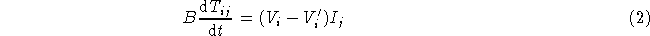in which B is a time constant and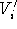is the feedback learning signal as described in the following.

The feedback learning signalis generated by a Hopfield type associative memory network: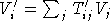, in which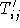is the strength of the associative connection from neuron j to neuron i, which is the recent correlation between the neuronal activities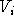and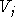determined by Hebbian learning with a decay term [5,6,10]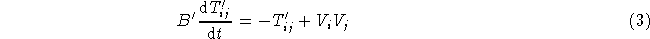in which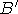is a time constant. Theandare only involved in learning and do not directly affect the network outputs.Next: Dynamic Stability Up: Associative Decorrelation Dynamics Previous: Associative Decorrelation Dynamics